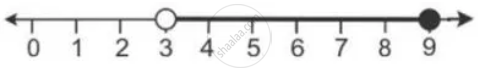Share

Solve and Graph the Solution Set Of: 5 > P – 1 > 2 Or 7 ≤ 2p – 1 ≤ 17, P ∈ R - ICSE Class 10 - Mathematics

ConceptLinear Inequations in One Unknown

Question

Solve and graph the solution set of:

5 > p – 1 > 2 or 7 ≤ 2p – 1 ≤ 17, p ∈ R

Solution

5 > p – 1 > 2 or 7 ≤ 2p – 1 ≤ 17

6 > p > 3 or 8 ≤ 2p ≤ 18

6 > p > 3 or 4 ≤ p ≤ 9

Graph of solution set of 6 > p > 3 or 4 ≤ p ≤ 9

= Graph of points which belong to 6 > p > 3 or 4 ≤ p ≤ 9 or both

= Graph of points which belong to 3 < p ≤ 9

Thus, the graph of the solution set is:Is there an error in this question or solution?

APPEARS IN

Solution Solve and Graph the Solution Set Of: 5 > P – 1 > 2 Or 7 ≤ 2p – 1 ≤ 17, P ∈ R Concept: Linear Inequations in One Unknown.
S